Click to Chat

1800-1023-196

+91-120-4616500

CART 0

• 0

MY CART (5)

Use Coupon: CART20 and get 20% off on all online Study Material

ITEM
DETAILS
MRP
DISCOUNT
FINAL PRICE
Total Price: Rs.

There are no items in this cart.
Continue Shopping• Complete Physics Course - Class 11
• OFFERED PRICE: Rs. 2,968
• View Details

Fluid Dynamics

Table of Content

Bernoulli's Theorem

Bernoulli's Principle is used in the following and many other ways

Action of Atomizer

Taking off of an Aeroplane

Velocity of Efflux

Regarding Viscosity the following are Worth Noting

Related Resources

Bernoulli's Theorem“Bernoulli's principle thus says that a rise (fall) in pressure in a flowing fluid must always be accompanied by a decrease (increase) in the speed, and conversely, if an increase (decrease) in , the speed of the fluid results in a decrease (increase) in the pressure”.

Consider a tube of flow, ABCD. In a time Δt, liquid moves and the liquid element becomes A'B'C'D'. In other words, we can also interpret that ABB'A' has gone to DCC'D'.Δm = ρA1v1 Δt = ρ A2v2 Δt

Work done by fluid pressure at end 1 = (P1A1)v1 Δt = P1 Δm/r

Work done by fluid pressure at end 2 = -(P2A2)v2 Δt = -P2 Δm/r

Work done by gravity = -(Δm)g(h2 - h1)

Change in kinetic energy = (1/2) Δm [v22 - v12]

Using, work-energy theorem (W = ΔK),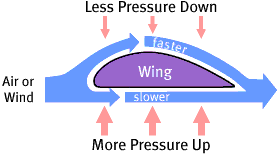P1 Δm/ρ - P2 Δm/ρ - Δmg (h2 - h1) = 1/2 Δm [V22 - V12]

Or  p1/ρ + gh1 + V12/2 = P2 + gh2 + V22/2

Or  P1 + ρgh1 + ρV12/2 = P2 + ρgh2 + ρV22/2

Or,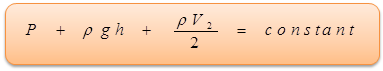In a streamline flow of an ideal fluid, the sum of pressure energy per unit volume, potential energy per unit volume and kinetic energy per unit volume is always constant at all cross sections of the liquid.

Bernoulli's equation is valid only for incompressible steady flow of a fluid with no viscosity.

A raft of wood of mass 120 kg floats in water. The weight that can be put on the raft to make it just sink is (10x) kg. Find value of x. Given density of raft = 600 kg/m3.

Solution

(mraft + m)g = Vraft ρwg

Thus, m = Vraftρw – mraft

= [(120/600)1000] – 120

= 80 kg

Illustration

The figure shows how the stream of water emerging from a faucet necks down as it falls. The area changes from A0 to A through a fall of h. At what does the water flow the top tap?Solution

Equation of continuity: A0 V0 = AV

Bernoulli equation:P0 + ρgh + 1/2 ρ v02 + ρg(0) + 1/2 ρv2

Solving for v0, v0 = √((2ghA2 A02)/(A02-A2 ))

= (AA0 √2gh)/√(A02-A2 ).

Note: As the jet is going into the atmosphere, the pressure at A and A0 are equal to the atmospheric pressure.

Illustration

Water enters a house through a pipe with inlet diameter of 2.0 cm at an absolute pressure of 4.0 × 105 Pa (about 4 atm). A 1.0 cm diameter pipe leads to the second floor bathroom 5.0 m above. When flow speed at the inlet pipe is 1.5 m/s, find the flow speed, pressure and volume flow rate in the bathroom.

Solution

Let points 1 and 2 be at the inlet pipe and at the bathroom, then from continuity equation,

a1v1 = a2v2     => v2 = 6.0 m/s

Now, applying Bernoulli's equation at the inlet (y = 0) and at the bathroom (y2 = 5.0 m).

As p + 1/2 σv2 + σgy = constant

Hence, p2 = p1 - 1/2 ρ(v22-v12 ) - ρg(y2-y1 )

which gives p2 = 3.3 × 105 Pa

The volume flow rate = A2v2 = A1v1 = Π/4 (0.1)26 = 4.7 × 10-4 m3/s.

Illustration

Water coming out of jet having across sectional area a, with a speed v strikes a stationary plate and stops after striking. Find the force exerted by the water jet on the plate.

Solution

The change of momentum of water in time dt = 0 - ρav2dt î = -ρav2dt î where is a unit vector in the direction of the velocity of the jet. The rate of change of momentum of water jet = -ρav2 î.

Thus the force exerted on the water jet by the plate = -ρav2 î

The force exerted on the plate by the water jet = ρav2 î .

Enquiry: Can the in-swing and out-swing of a Cricket ball be explained by this principle?

Yes, when a spinning ball is thrown, it deviates from its usual path in flight. This effect is called Magnus effect and plays an important role in tennis, cricket etc. By applying appropriate spin the moving ball can be made to deviate in any desired direction.

If a ball is moving from left to right and is also spinning about a horizontal axis perpendicular to the direction of motion, the resultant velocity of air above the ball will be (V + r ω) while below the ball (V-rω). So in accordance with the Bernoulli's principle pressure above the ball will be lesser than that below it. Due to this difference of pressure upward force will act on the ball and hence, the ball will deviate from its usual path and will touch the ground following a path which curves less sharply, prolonging the flight.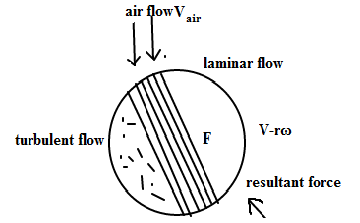Similarly if the spin is clockwise i.e. the ball is thrown with topspin, the force due to pressure difference will act in the direction of gravity and the ball will pitch more sharply shortening the flight.

Further more, if the ball is spinning about a vertical axis, the curving will be side ways producing the so-called out-swing or in-swing.

The following phenomena can also be explained using Bernoulli's equation.

(a) Drop of pressure when a fluid from broader to a narrower horizontal pipe. We have Av = constant.

Thus, When area is large, velocity is small and vice-versa.

But by Bernoulli's equation,

P + 1/2ρv2 = constant for a horizontal pipe. i.e. When velocity is large pressure is small and vice-versa. Thus, pressure at smaller cross-area is lesser.

(b) Blowing off, of roofs of windstorms

During a tornado or a hurricane, when a high-speed wind blows over a straw or tin roof, it creates low pressure above it, in accordance with Bernoulli's principle. However the pressure under the roof is still atmospheric. So due to this difference of pressure the roof is lifted up and is then blown off by the wind.

(c) Attraction between two closely parallel moving boats.

When two boats are moving in the same direction the water or air between them move faster than that on the remote sides. Consequently, in accordance with the Bernoulli equation, the pressure between them is reduced. Hence due to pressure difference they are pulled towards each other creating the so-called attraction.

Enquiry: What is the use of Bernoulli's principle in practice?

Bernoulli's Principle is used in the following and many other ways

(a) Action of Atomizer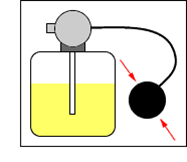The action of aspirator, carburetor, paint gun, scent spray or insect sprayer is based on Bernoulli's principle. In all these, by means of motion of piston r in a cylinder C, high speed air is passed over a tube T, dipped in a liquid L to be sprayed.High speed air creates low pressure over the tube due to which liquid rise in it and is then blown off with expanded air.

(b) Taking off of an Aeroplane

This is also based on Bernoulli's Principle. The wings of aeroplane are made tapering. Due to this specific shape of wings when aeroplane runs, air pases at high speed over it as compared to lower surface. This difference of air speeds above and below the wings, in accordance with the Bernoulli's principle, creates a pressure difference, which increases with the speed of the aeroplane due to which an upward force called dynamic lift (= pressure difference x area of wing) acts on the plane. When this lift becomes greater than the weight of the plane, the plane rises up.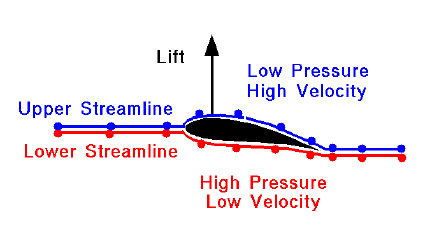(c) Velocity of Efflux

Liquid is filled in a vessel up to a height H and a hole is made at a depth h below the free surface of the liquid as shown. Taking the level of hole as reference level and applying Bernoulli's principle to the liquid just inside and outside the hole (assuming the liquid to be at rest inside), we get,

P0 - hpg - 0 = P0 - 1/2ρv2

Or  v = √2gh [Which is also known as Toricelli's Theorem].

Refer this video for more about Bernoulli's Principle

Enquiry: Where do we see the application of Bernoulli's theorem?

Yes, Bernoulli's theorem finds application in many devices, two of which are described below.

(a) Venturimeter

This is an instrument for measuring velocity of flow of liquids & gases [fluids].

The instrument is connected horizontally in the tube in which the rate of flow is to be measured. If PA is pressure at A & PB is pressure at B. PA-PB = hrg [h is difference of heights of liquids of density r is vertical tubes].

Let V1 = Velocity at A

V2 = Velocity at B.

Now, A1 V1 = A2 V2.

Let us first consider the case of liquids. If the tube is held horizontal, then by application of Bernoulli's theorem,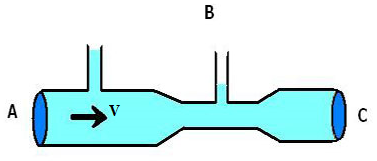PA/ρ+ (v12)/2 = PB/ρ + (v22)/2

=> v22 – v12 = 2/ρ (PA - PB )= (2/ρ) hρg = 2hg

=> Q2/(A22 ) - Q2/(A12 ) = 2gh, [?Q=Av]

where Q = amount of liquid flowing in or flowing out per unit time.

=> Q = A1 A2 √(2gh/(A12 - A22 ))

As Q = A1V1 = A2V2,

Hence V1 and V2 can be calculated.

Explanation the velocity is measured using this formula.

For gases, let d be the density of the gas,

PA - PB = hrg

from Bernoulli's Equation,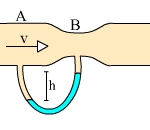PA/d+ (V12)/2 = PB/d + (V22)/2

=> v22 – v12 = 2/d (PA - PB )

=> v22 – v12 = 2/d (PA - PB )hρg

=> Q2/(A22 ) - Q2/(A12 ) = (2/d) hρg

=> Q = A1 A2 √(2hρg/(d(A12 - A22)))

(b) Pilot TubeLike Venturimeter, a pilot tube is also used for measuring flow rates of fluids.

For liquids, if A & B are in the same horizontal plane explain the figure. Applying Bernoulli's Equation,

PA/ρ +  1/2 v2 =  PB/ρ + 0

or 2(PB - PA )/P = v2

or v = √(2(PB - PA )/ρ) = √2gh

[? (PB - PA ) = hρg]

Q = Av = A√2gh.

For gases, Let r be the density of the liquid in vertical tube,

PB - PA = hrg.

Applying Bernoulli's theorem at A & B,

PA + 1/2 dv2= PB

=>  v2 =  2(PA - PB )/d

=>  v = √(2hρg/d)

Then      Q = A√(2hρg/d).

Enquiry: Considering the motion of fluids, do the fluid streamlines encounter any opposition?

If you recall, while studying critical velocity we talked about something called viscosity.

The property of a fluid due to which it opposes the relative motion between its consecutive layers is called viscosity and the force between the layers opposing the motion is called viscous force.

According to Newton, the viscous force F acting on any layer of a fluid is directly proportional to its area and the velocity gradient across the layer i.e.

Where A is the area of the layers, dv/dy is velocity gradient and η is called the coefficient of viscosity. The negative sign shows that viscous force acts in a direction opposite to that of the flow of fluid. Coefficient of viscosity depends only on the nature of the fluid and is independent of area considered. Its dimensions are [ML-1 T-1].

Regarding Viscosity the following are Worth Noting

(i) Viscosity of liquids is much greater than gases.

(ii) With rise in temperature the viscosity of the liquids decreases while that for gases increases.

(iii) In case of liquids viscosity increases with density while for gases it decreases with increase in density.

(iv) With increase in pressure, the viscosity of liquids (except water) increases while that of gases is practically independent of pressure.

(v)  From kinetic theory, viscosity represents transport of momentum, i.e. viscosity is due to the difference in the momenta of two layers.

(vi) In case of steady flow of a liquid of viscosity η in a cylindrical tube of length L and radius r under a pressure difference r across it, the velocity of flow at a distance y from the axis is given by v = ρ/4ηL (r2 - y2 ) The velocity profile is a parabola. On x-axis y=0

=> v = pr2/4ηL

(vii) In case of a steady flow of a viscous liquid in a cylindrical tube of length L and radius r under a pressure difference p across it, the volume of liquid flowing per second is given by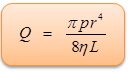This is Poiseuille's Formula.

Think:When we throw a dense object in a fluid the force of buoyancy cannot balance the force of gravity and then the object accelerates downwards. Had it been an ideal fluid, then it would have accelerated indefinitely till it reached the bottom but due to relative motion between object and fluid, resistive (viscous) forces come into play.The fluid is homogeneous.

The viscous fluid is indefinite in extent.

Spherical body is perfectly rigid and smooth.

The viscous drag F is independent of the relative velocity between different layers of liquid. It depends only on velocity gradient within the liquid.

Friction is there between two bodies of different natures while viscous force is in between two layers of same liquid.

Force of friction is independent of relative velocity while force of  viscosity depends upon velocity gradient.Question 1

In case of streamlined flow of liquid, the loss energy is:

(a) minimum              (b) maximum

(c) zero                     (d) infinite

Question 2

A flowing liquid possesses:

(a) K.E                      (b) P.E

(c) pressure energy    (d) all the three

Question 3

With increase of temperature , the viscosity of liquid and gases:

(a) increase for both                                                    (b) decrease for both

(c) increases for liquids and decreases for gases          (d) decrease for liquids and increase for gases

Question 4

The velocity of falling rain drops attains limited value because of :

(a) upthrust of air               (b) viscous force exerted by air

(c) surface tension effects   (d) air currents in atmosphere.Q.1
Q.2
Q.3
Q.4

a

d

a

b

Related Resources

You might like to refer Fluid Statics.

For getting an idea of the type of questions asked, refer the  Previous Year Question Papers.### Course Features

• 101 Video Lectures
• Revision Notes
• Previous Year Papers
• Mind Map
• Study Planner
• NCERT Solutions
• Discussion Forum
• Test paper with Video Solution